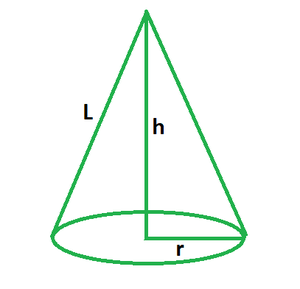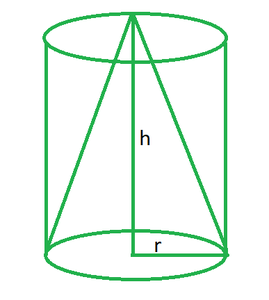# Volume of a Cone Formula

• Last Updated : 22 Mar, 2022

Mensuration is a branch of mathematics concerned with the study of various geometrical shapes. It also takes into account the areas and volumes of such geometrical forms. It is the branch of mathematics concerned with measurements, such as the articulation and application of algebraic equations to the measurement of areas, volumes, and other geometric form qualities, whereas geometry is the branch of mathematics concerned with spatial relationships.

### Cone

A cone is a solid three-dimensional geometric object with a circular base and a sharp edge at the top known as the apex. It has a single face and a single vertex. For a cone, there are no edges. It is a form with a curving top and a round base. A cone has one face, one vertex, and no edges. Its slant height is the length of the line segment from the peak of the cone to any point on the circle of the cone’s base. A right circular cone is one that has its peak directly above the circular base at a perpendicular distance. An oblique cone is one that does not have its apex directly above the circular base.### Volume of a Cone

A cone’s volume is defined as the amount of space or capacity it fills. The volume of a cone is measured in cubic units such as cm3, m3, in3, and so on. By rotating a triangle around any of its vertices, a cone can be produced. A cone is a solid three-dimensional form having a circular base. It’s got a curved surface. The perpendicular height is the distance from the base to the vertex. A cone may be divided into two types: right circular cones and oblique cones. The vertex of the right circular cone is vertically above the centre of the base, but the vertex of the oblique cone is not vertically above the centre of the base.

The formula for the volume of a cone is given by,

V =where,

r = radius of the cone,

h = height of the cone,

π = 22/7

Also, the relationship between the cone’s volume and slant height by applying Pythagoras’ theorem to it is given by,

h2 + r2 = L2

=> h = √(L2 – r2)

Hence, the volume of cone in terms of its slant height is given by,

V =Derivation

Suppose we have a cone with a circular base whose radius is r and height is h.We know that the volume of a cone is equal to one-third of the volume of a cylinder having the same base radius and height.

So, the volume becomes,

V = 1/3 x Circular Base area x Height

= 1/3 x πr2 x h

=  πr2h/3

This derives the formula for volume of cone.

### Sample Questions

Question 1. Find the volume of a cone for the radius of 7 cm and height of 14 cm.

Solution:

We have, r = 7 and h = 14.

Volume of cone = 1/3 πr2h

= (1/3) (22/7) (7) (7) (14)

= (1/3) (7) (7) (2)

= 32.66 cm3

Question 2. Find the volume of a cone for the radius of 5 cm and height of 9 cm.

Solution:

We have, r = 5 and h = 9.

Volume of cone = 1/3 πr2h

= (1/3) (3.14) (5) (5) (9)

= (3.14) (5) (5) (3)

= 235.49 cm3

Question 3. Find the volume of a cone for the radius of 7 cm and height of 12 cm.

Solution:

We have, r = 7 and h = 12.

Volume of cone = 1/3 πr2h

= (1/3) (22/7) (7) (7) (12)

= (22) (7) (4)

= 616 cm3

Question 4. Find the volume of a cone for the radius of 8 cm and height of 15 cm.

Solution:

We have, r = 8 and h = 15.

Volume of cone = 1/3 πr2h

= (1/3) (22/7) (8) (8) (15)

= (1/3) (22/7) (8) (8) (5)

= 335.02 cm3

Question 5. Find the volume of a cone for the diameter of 24 cm and slant height of 13 cm.

Solution:

We have, 2r = 24

=> r = 24/2

=> r = 12

Also, l = 13.

Volume of cone = 1/3 πr2 √(l2 – r2)

= (1/3) (22/7) (12) (12) (√(132 – 122)

= (1/3) (22/7) (12) (12) (5)

= 754.28 cm3

Question 6. Find the volume of a cone for the diameter of 16 cm and slant height of 10 cm.

Solution:

We have, 2r = 16

=> r = 16/2

=> r = 8

Also, l = 10.

Volume of cone = 1/3 πr2 √(l2 – r2)

= (1/3) (22/7) (8) (8) (√(102 – 82)

= (1/3) (22/7) (8) (8) (6)

= 402.048 cm3

Question 7. Find the volume of a cone for the height of 8 cm and slant height of 17 cm.

Solution:

We have h = 8 and l = 10.

Find the value of r.

r = √(l2 – h2)

= √(172 – 82)

= √(289 – 64)

= 15

Volume of cone = 1/3 πr2h

= (1/3) (22/7) (15) (15) (8)

= (1/3) (22/7) (5) (15) (8)

= 1884.6 cm3

My Personal Notes arrow_drop_up
Recommended Articles
Page :